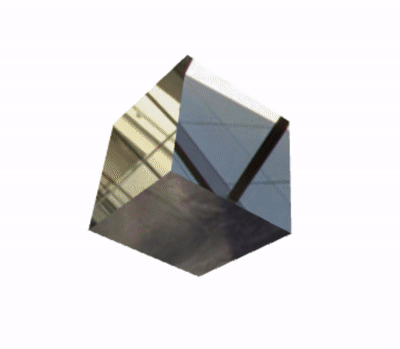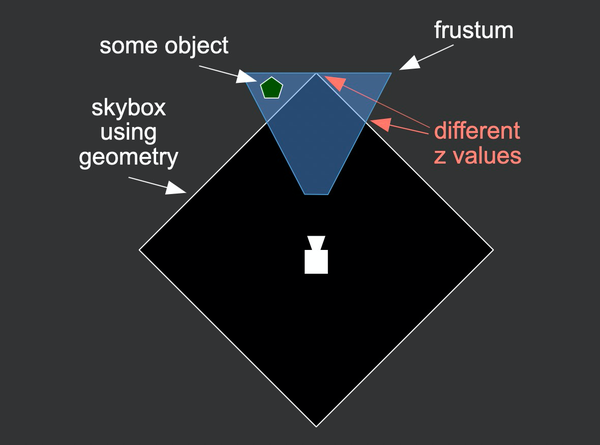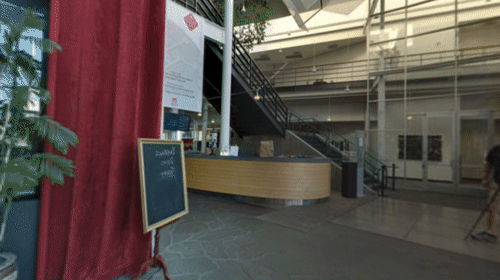## 立方体纹理的应用

• 正方体表面贴图
• 用于环境贴图（反射贴图），模拟镜面反射结果
• 天空盒

### 立方体环境贴图### 天空盒$P_{NDC} = M_{projection} * M_{view} * M_{model} * P$

$P_{world} = (M_{projection} * M_{view})^{-1} * P_{NDC}$